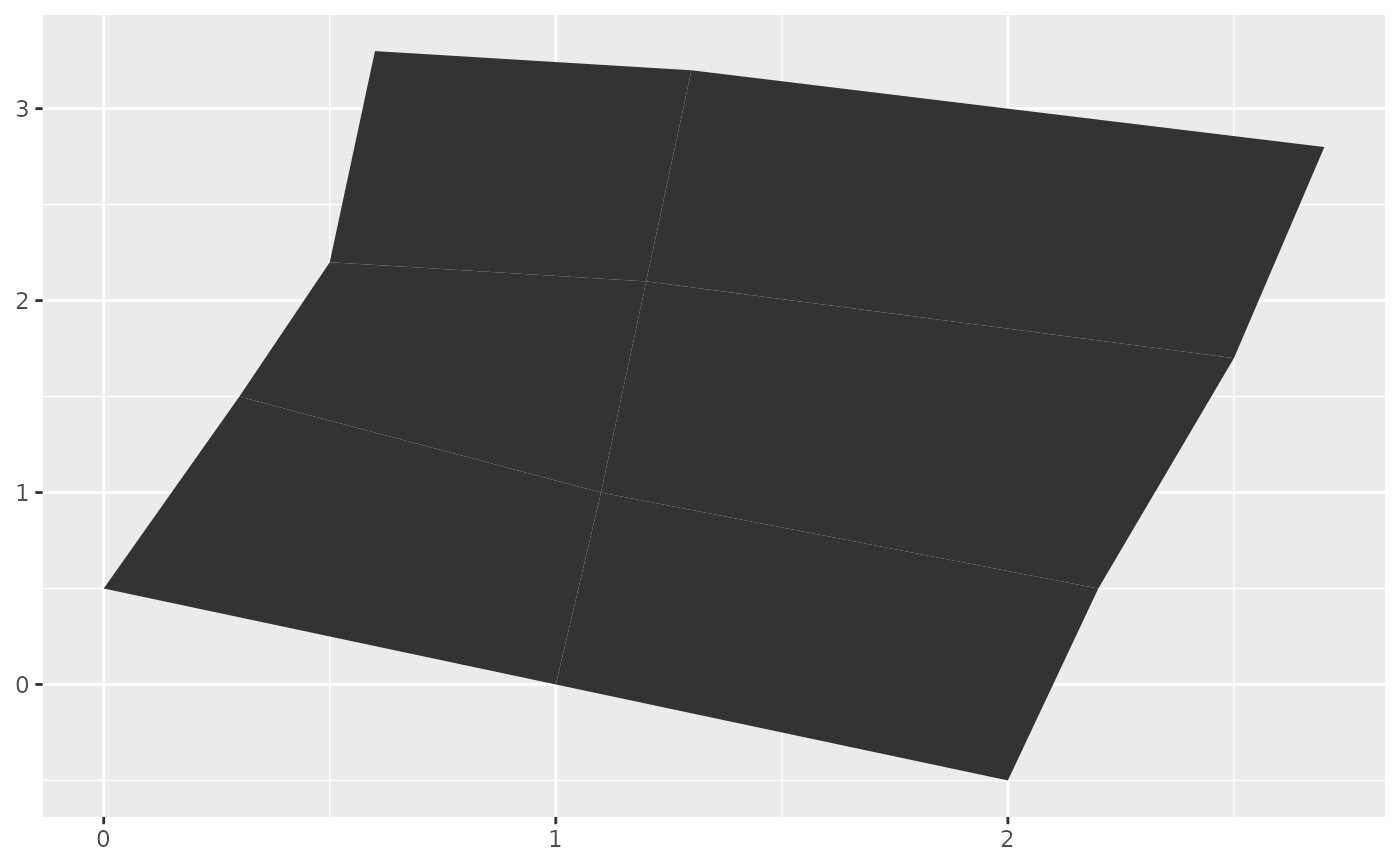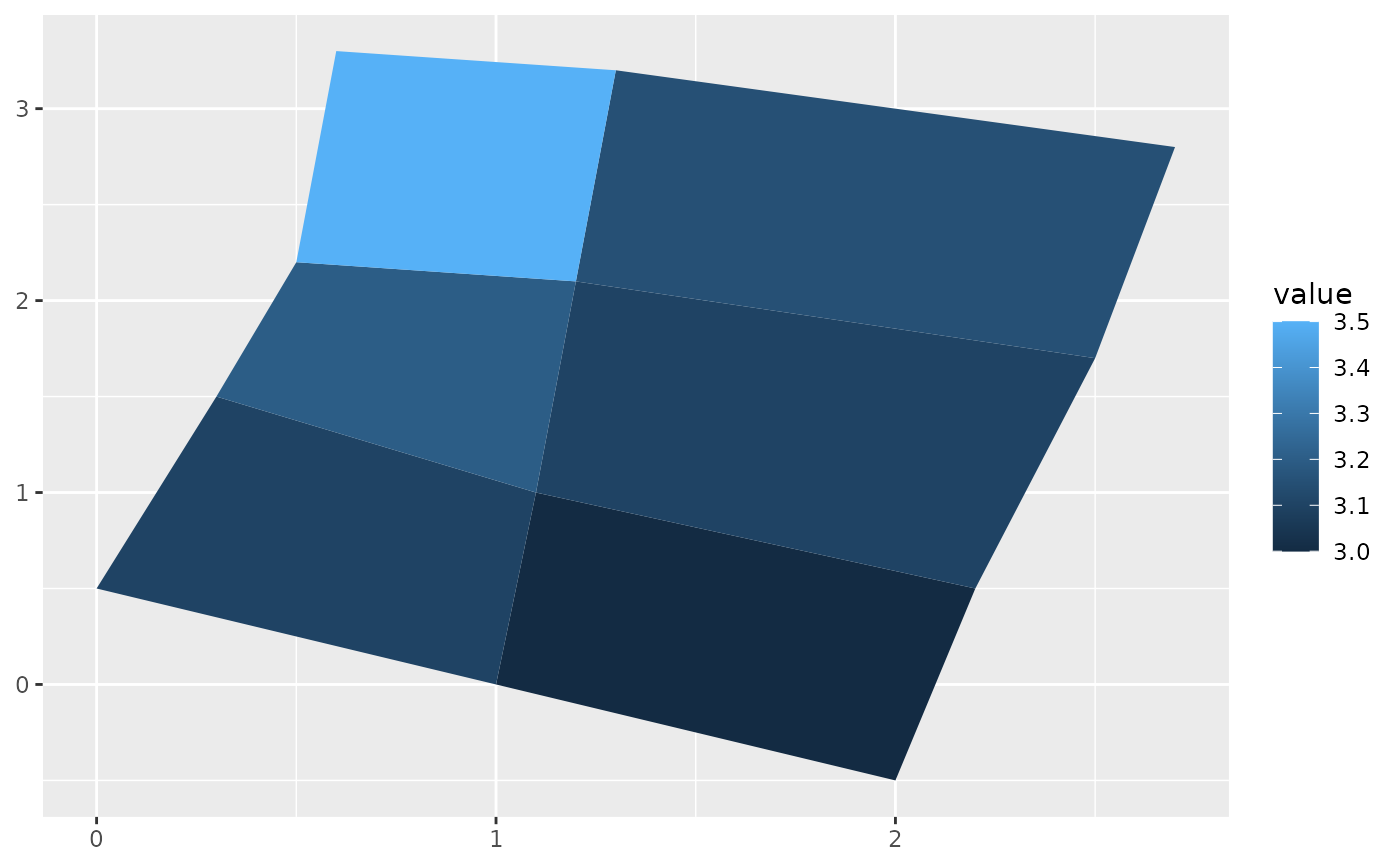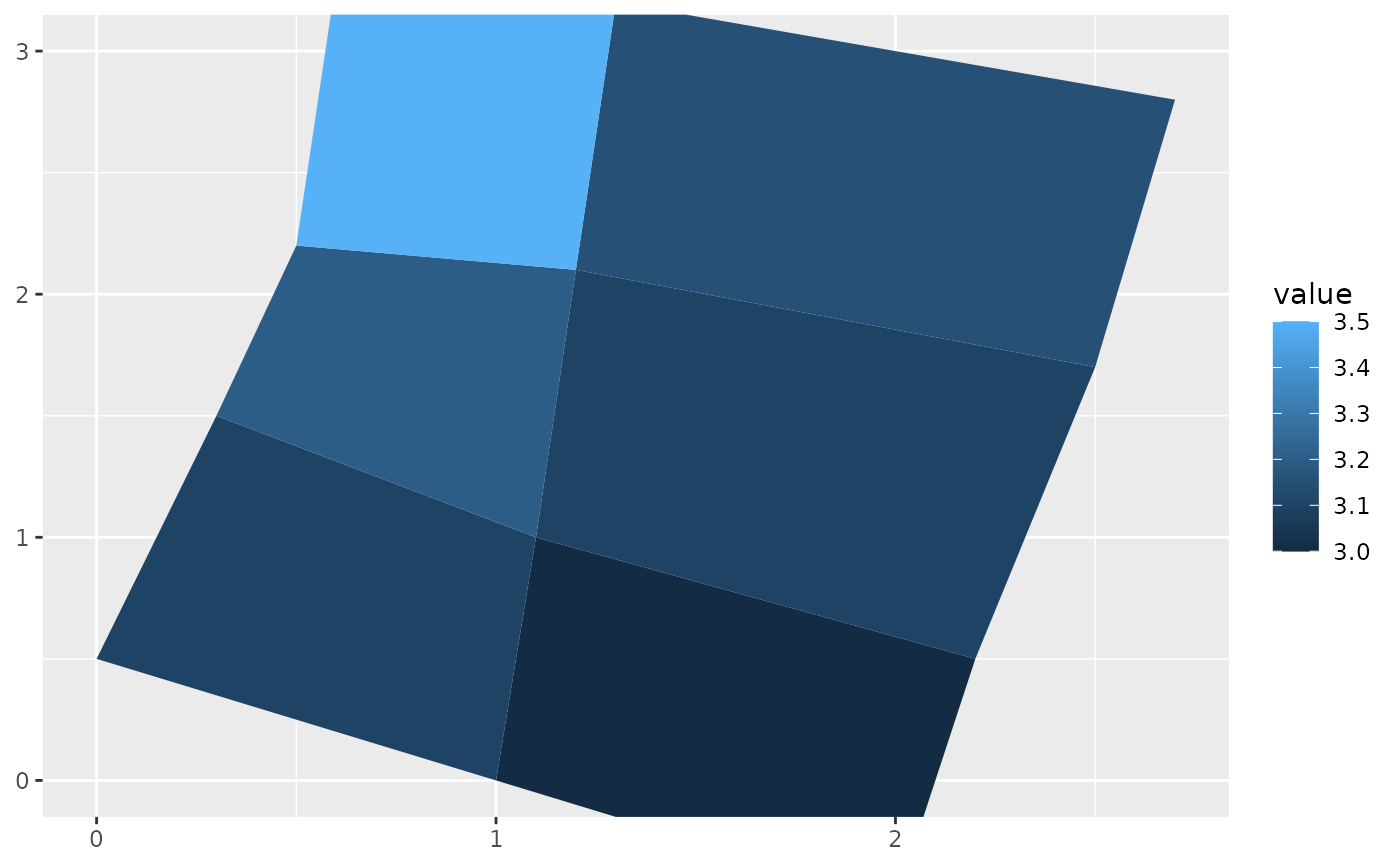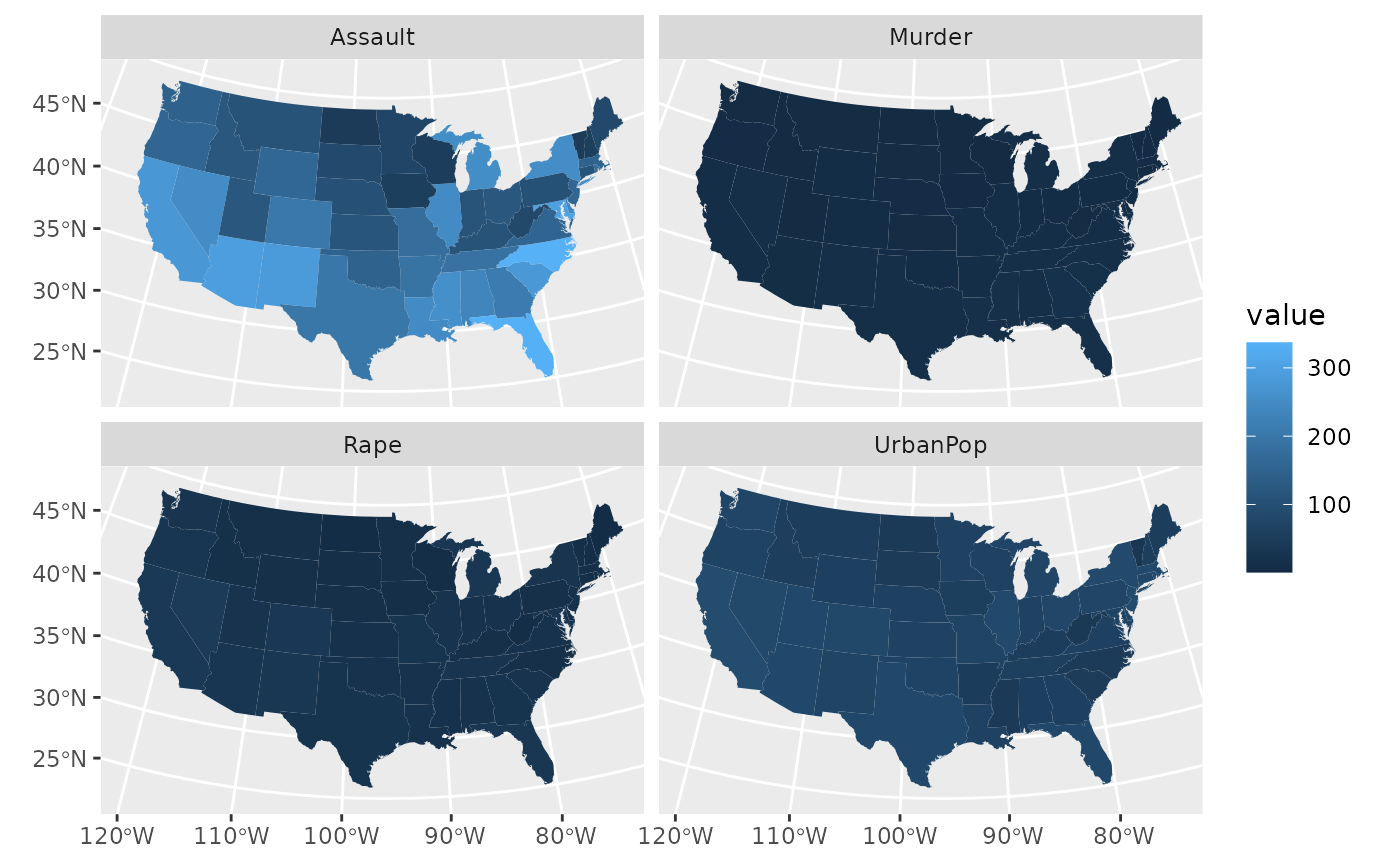This is pure annotation, so does not affect position scales.

## Usage

geom_map(
mapping = NULL,
data = NULL,
stat = "identity",
...,
map,
na.rm = FALSE,
show.legend = NA,
inherit.aes = TRUE
)

## Arguments

mapping

Set of aesthetic mappings created by aes() or aes_(). If specified and inherit.aes = TRUE (the default), it is combined with the default mapping at the top level of the plot. You must supply mapping if there is no plot mapping.

data

The data to be displayed in this layer. There are three options:

If NULL, the default, the data is inherited from the plot data as specified in the call to ggplot().

A data.frame, or other object, will override the plot data. All objects will be fortified to produce a data frame. See fortify() for which variables will be created.

A function will be called with a single argument, the plot data. The return value must be a data.frame, and will be used as the layer data. A function can be created from a formula (e.g. ~ head(.x, 10)).

stat

The statistical transformation to use on the data for this layer, as a string.

...

Other arguments passed on to layer(). These are often aesthetics, used to set an aesthetic to a fixed value, like colour = "red" or size = 3. They may also be parameters to the paired geom/stat.

map

Data frame that contains the map coordinates. This will typically be created using fortify() on a spatial object. It must contain columns x or long, y or lat, and region or id.

na.rm

If FALSE, the default, missing values are removed with a warning. If TRUE, missing values are silently removed.

show.legend

logical. Should this layer be included in the legends? NA, the default, includes if any aesthetics are mapped. FALSE never includes, and TRUE always includes. It can also be a named logical vector to finely select the aesthetics to display.

inherit.aes

If FALSE, overrides the default aesthetics, rather than combining with them. This is most useful for helper functions that define both data and aesthetics and shouldn't inherit behaviour from the default plot specification, e.g. borders().

## Aesthetics

geom_map() understands the following aesthetics (required aesthetics are in bold):

• map_id

• alpha

• colour

• fill

• group

• linetype

• size

• subgroup

Learn more about setting these aesthetics in vignette("ggplot2-specs").

## Examples

# When using geom_polygon, you will typically need two data frames:
# one contains the coordinates of each polygon (positions),  and the
# other the values associated with each polygon (values).  An id
# variable links the two together

ids <- factor(c("1.1", "2.1", "1.2", "2.2", "1.3", "2.3"))

values <- data.frame(
id = ids,
value = c(3, 3.1, 3.1, 3.2, 3.15, 3.5)
)

positions <- data.frame(
id = rep(ids, each = 4),
x = c(2, 1, 1.1, 2.2, 1, 0, 0.3, 1.1, 2.2, 1.1, 1.2, 2.5, 1.1, 0.3,
0.5, 1.2, 2.5, 1.2, 1.3, 2.7, 1.2, 0.5, 0.6, 1.3),
y = c(-0.5, 0, 1, 0.5, 0, 0.5, 1.5, 1, 0.5, 1, 2.1, 1.7, 1, 1.5,
2.2, 2.1, 1.7, 2.1, 3.2, 2.8, 2.1, 2.2, 3.3, 3.2)
)

ggplot(values) +
geom_map(aes(map_id = id), map = positions) +
expand_limits(positions)ggplot(values, aes(fill = value)) +
geom_map(aes(map_id = id), map = positions) +
expand_limits(positions)ggplot(values, aes(fill = value)) +
geom_map(aes(map_id = id), map = positions) +
expand_limits(positions) + ylim(0, 3)# Better example
if (require(maps)) {

crimes <- data.frame(state = tolower(rownames(USArrests)), USArrests)

# Equivalent to crimes %>% tidyr::pivot_longer(Murder:Rape)
vars <- lapply(names(crimes)[-1], function(j) {
data.frame(state = crimes$state, variable = j, value = crimes[[j]]) }) crimes_long <- do.call("rbind", vars) states_map <- map_data("state") ggplot(crimes, aes(map_id = state)) + geom_map(aes(fill = Murder), map = states_map) + expand_limits(x = states_map$long, y = states_map$lat) last_plot() + coord_map() ggplot(crimes_long, aes(map_id = state)) + geom_map(aes(fill = value), map = states_map) + expand_limits(x = states_map$long, y = states_map\$lat) +
facet_wrap( ~ variable)
}